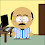### Prime Numbers Generator

did u know that 34136029 is a prime number?!!
well.. that's what i recently realized :)

I thought of writing a piece of code for that purpose.. wondering how far i can get.

The idea of the code is simple
We know that 2 and 3 are prime numbers
the code checks every following odd numbers to be prime or not.

To verify a prime number, the number divisibility by the prime numbers generated so far is checked. (29 is a prime number.. because it id not divisible by 2, 3, 5, 7, 11, 13, 17, 19 nor 23)

We only need to verify divisibility against the numbers smaller than or equal to the root of the number in hand. (We needn't check the divisibility of 33 with 11, as we already must have found out it is divisible by 3)..

I did not want to go with the mathematical root evaluation as i am not sure about its load. I used bits handling instead. the root of a number uses at most half of the number of bits that the original number uses.

i only made a naive java implementation so far. download the jar file and try it yourself "java -jar prime-generator.jar"

Here i explain the implementation

Generating Primes
This method starts by marking 2 and 3 as primes.. then check the rest of the odd numbers until the upper limit is reached (this is a value passed as a parameter to the class OR the largest number that can be held in a long primitive type)The longest long value can be found my shifting 1's to fill the bits of a long variable before it converts to a negative number.to verify a prime number, the number in hand is compared to the identified prime numbers that are less than the assumed root limit.Instead of evaluating the mathematical root. i used that fact that the root of a number will use at most half the number of bits used by that number.the output for the program is

1: 2
2: 3
3: 5
.
.
.
485785: 7143883
485786: 7143887
485787: 7143911
485788: 7143931
485789: 7143959
.
.
.
2097148: 34135967
2097149: 34135999
2097150: 34136009
2097151: 34136021
2097152: 34136029

the code generated 2097152 prime numbers.. starting with 2 and ending with 34136029.

i think i can go farther...

it was stopped by ArrayIndexOutOfBoundsException. there must be a way to get a larger array... or i have to find another way to track the numbers instead of having them in memory.Anonymous said…